# 6.2 Graphs of exponential functions  (Page 6/6)

 Page 6 / 6

## Verbal

What role does the horizontal asymptote of an exponential function play in telling us about the end behavior of the graph?

An asymptote is a line that the graph of a function approaches, as $\text{\hspace{0.17em}}x\text{\hspace{0.17em}}$ either increases or decreases without bound. The horizontal asymptote of an exponential function tells us the limit of the function’s values as the independent variable gets either extremely large or extremely small.

What is the advantage of knowing how to recognize transformations of the graph of a parent function algebraically?

## Algebraic

The graph of $\text{\hspace{0.17em}}f\left(x\right)={3}^{x}\text{\hspace{0.17em}}$ is reflected about the y -axis and stretched vertically by a factor of $\text{\hspace{0.17em}}4.\text{\hspace{0.17em}}$ What is the equation of the new function, $\text{\hspace{0.17em}}g\left(x\right)?\text{\hspace{0.17em}}$ State its y -intercept, domain, and range.

$g\left(x\right)=4{\left(3\right)}^{-x};\text{\hspace{0.17em}}$ y -intercept: $\text{\hspace{0.17em}}\left(0,4\right);\text{\hspace{0.17em}}$ Domain: all real numbers; Range: all real numbers greater than $\text{\hspace{0.17em}}0.$

The graph of $\text{\hspace{0.17em}}f\left(x\right)={\left(\frac{1}{2}\right)}^{-x}\text{\hspace{0.17em}}$ is reflected about the y -axis and compressed vertically by a factor of $\text{\hspace{0.17em}}\frac{1}{5}.\text{\hspace{0.17em}}$ What is the equation of the new function, $\text{\hspace{0.17em}}g\left(x\right)?\text{\hspace{0.17em}}$ State its y -intercept, domain, and range.

The graph of $\text{\hspace{0.17em}}f\left(x\right)={10}^{x}\text{\hspace{0.17em}}$ is reflected about the x -axis and shifted upward $\text{\hspace{0.17em}}7\text{\hspace{0.17em}}$ units. What is the equation of the new function, $\text{\hspace{0.17em}}g\left(x\right)?\text{\hspace{0.17em}}$ State its y -intercept, domain, and range.

$g\left(x\right)=-{10}^{x}+7;\text{\hspace{0.17em}}$ y -intercept: $\text{\hspace{0.17em}}\left(0,6\right);\text{\hspace{0.17em}}$ Domain: all real numbers; Range: all real numbers less than $\text{\hspace{0.17em}}7.$

The graph of $\text{\hspace{0.17em}}f\left(x\right)={\left(1.68\right)}^{x}\text{\hspace{0.17em}}$ is shifted right $\text{\hspace{0.17em}}3\text{\hspace{0.17em}}$ units, stretched vertically by a factor of $\text{\hspace{0.17em}}2,$ reflected about the x -axis, and then shifted downward $\text{\hspace{0.17em}}3\text{\hspace{0.17em}}$ units. What is the equation of the new function, $\text{\hspace{0.17em}}g\left(x\right)?\text{\hspace{0.17em}}$ State its y -intercept (to the nearest thousandth), domain, and range.

The graph of $\text{\hspace{0.17em}}f\left(x\right)=2{\left(\frac{1}{4}\right)}^{x-20}$ is shifted left $\text{\hspace{0.17em}}2\text{\hspace{0.17em}}$ units, stretched vertically by a factor of $\text{\hspace{0.17em}}4,$ reflected about the x -axis, and then shifted downward $\text{\hspace{0.17em}}4\text{\hspace{0.17em}}$ units. What is the equation of the new function, $\text{\hspace{0.17em}}g\left(x\right)?\text{\hspace{0.17em}}$ State its y -intercept, domain, and range.

$g\left(x\right)=2{\left(\frac{1}{4}\right)}^{x};\text{\hspace{0.17em}}$ y -intercept: Domain: all real numbers; Range: all real numbers greater than $\text{\hspace{0.17em}}0.$

## Graphical

For the following exercises, graph the function and its reflection about the y -axis on the same axes, and give the y -intercept.

$f\left(x\right)=3{\left(\frac{1}{2}\right)}^{x}$

$g\left(x\right)=-2{\left(0.25\right)}^{x}$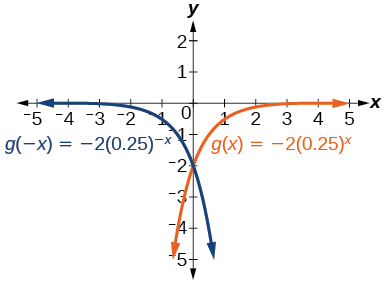y -intercept: $\text{\hspace{0.17em}}\left(0,-2\right)$

$h\left(x\right)=6{\left(1.75\right)}^{-x}$

For the following exercises, graph each set of functions on the same axes.

$f\left(x\right)=3{\left(\frac{1}{4}\right)}^{x},$ $g\left(x\right)=3{\left(2\right)}^{x},$ and $\text{\hspace{0.17em}}h\left(x\right)=3{\left(4\right)}^{x}$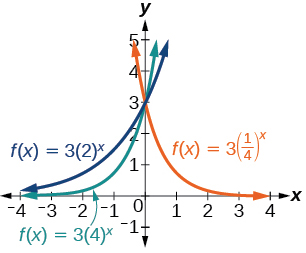$f\left(x\right)=\frac{1}{4}{\left(3\right)}^{x},$ $g\left(x\right)=2{\left(3\right)}^{x},$ and $\text{\hspace{0.17em}}h\left(x\right)=4{\left(3\right)}^{x}$

For the following exercises, match each function with one of the graphs in [link] .

$f\left(x\right)=2{\left(0.69\right)}^{x}$

B

$f\left(x\right)=2{\left(1.28\right)}^{x}$

$f\left(x\right)=2{\left(0.81\right)}^{x}$

A

$f\left(x\right)=4{\left(1.28\right)}^{x}$

$f\left(x\right)=2{\left(1.59\right)}^{x}$

E

$f\left(x\right)=4{\left(0.69\right)}^{x}$

For the following exercises, use the graphs shown in [link] . All have the form $\text{\hspace{0.17em}}f\left(x\right)=a{b}^{x}.$

Which graph has the largest value for $\text{\hspace{0.17em}}b?$

D

Which graph has the smallest value for $\text{\hspace{0.17em}}b?$

Which graph has the largest value for $\text{\hspace{0.17em}}a?$

C

Which graph has the smallest value for $\text{\hspace{0.17em}}a?$

For the following exercises, graph the function and its reflection about the x -axis on the same axes.

$f\left(x\right)=\frac{1}{2}{\left(4\right)}^{x}$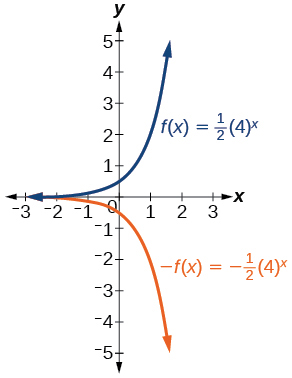$f\left(x\right)=3{\left(0.75\right)}^{x}-1$

$f\left(x\right)=-4{\left(2\right)}^{x}+2$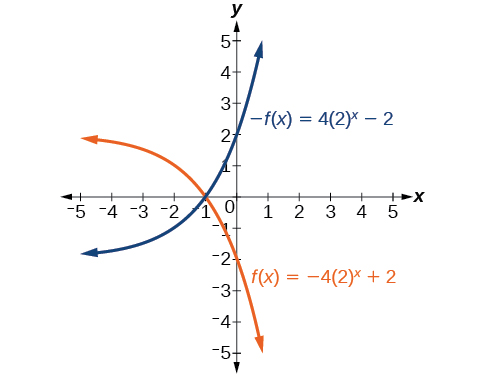For the following exercises, graph the transformation of $\text{\hspace{0.17em}}f\left(x\right)={2}^{x}.\text{\hspace{0.17em}}$ Give the horizontal asymptote, the domain, and the range.

$f\left(x\right)={2}^{-x}$

$h\left(x\right)={2}^{x}+3$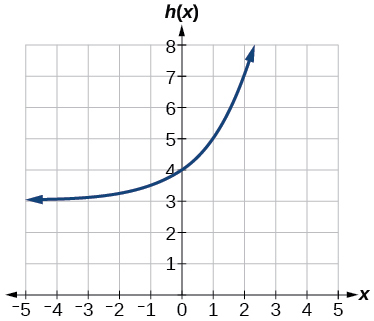Horizontal asymptote: $\text{\hspace{0.17em}}h\left(x\right)=3;$ Domain: all real numbers; Range: all real numbers strictly greater than $\text{\hspace{0.17em}}3.$

$f\left(x\right)={2}^{x-2}$

For the following exercises, describe the end behavior of the graphs of the functions.

$f\left(x\right)=-5{\left(4\right)}^{x}-1$

As $x\to \infty$ , $f\left(x\right)\to -\infty$ ;
As $x\to -\infty$ , $f\left(x\right)\to -1$

$f\left(x\right)=3{\left(\frac{1}{2}\right)}^{x}-2$

$f\left(x\right)=3{\left(4\right)}^{-x}+2$

As $x\to \infty$ , $f\left(x\right)\to 2$ ;
As $x\to -\infty$ , $f\left(x\right)\to \infty$

For the following exercises, start with the graph of $\text{\hspace{0.17em}}f\left(x\right)={4}^{x}.\text{\hspace{0.17em}}$ Then write a function that results from the given transformation.

Shift $f\left(x\right)$ 4 units upward

Shift $\text{\hspace{0.17em}}f\left(x\right)\text{\hspace{0.17em}}$ 3 units downward

$f\left(x\right)={4}^{x}-3$

Shift $\text{\hspace{0.17em}}f\left(x\right)\text{\hspace{0.17em}}$ 2 units left

Shift $\text{\hspace{0.17em}}f\left(x\right)\text{\hspace{0.17em}}$ 5 units right

$f\left(x\right)={4}^{x-5}$

Reflect $\text{\hspace{0.17em}}f\left(x\right)\text{\hspace{0.17em}}$ about the x -axis

Reflect $\text{\hspace{0.17em}}f\left(x\right)\text{\hspace{0.17em}}$ about the y -axis

$f\left(x\right)={4}^{-x}$

For the following exercises, each graph is a transformation of $\text{\hspace{0.17em}}y={2}^{x}.\text{\hspace{0.17em}}$ Write an equation describing the transformation.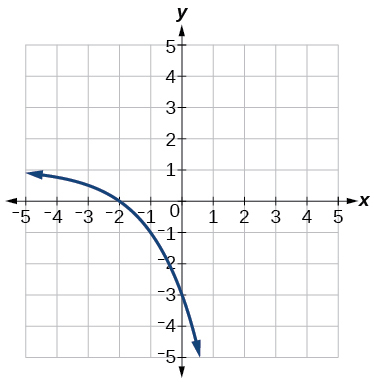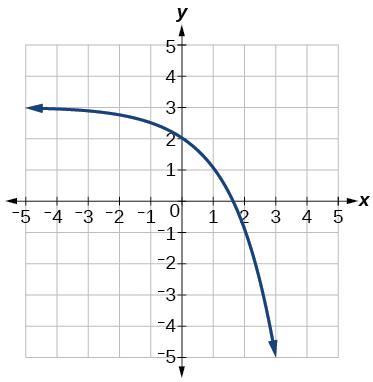$y=-{2}^{x}+3$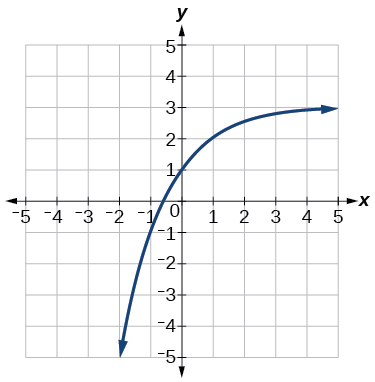For the following exercises, find an exponential equation for the graph.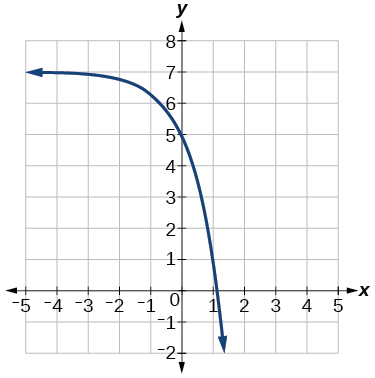$y=-2{\left(3\right)}^{x}+7$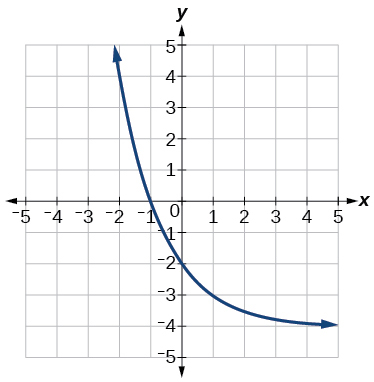## Numeric

For the following exercises, evaluate the exponential functions for the indicated value of $\text{\hspace{0.17em}}x.$

$g\left(x\right)=\frac{1}{3}{\left(7\right)}^{x-2}\text{\hspace{0.17em}}$ for $\text{\hspace{0.17em}}g\left(6\right).$

$g\left(6\right)=800+\frac{1}{3}\approx 800.3333$

$f\left(x\right)=4{\left(2\right)}^{x-1}-2\text{\hspace{0.17em}}$ for $\text{\hspace{0.17em}}f\left(5\right).$

$h\left(x\right)=-\frac{1}{2}{\left(\frac{1}{2}\right)}^{x}+6\text{\hspace{0.17em}}$ for $\text{\hspace{0.17em}}h\left(-7\right).$

$h\left(-7\right)=-58$

## Technology

For the following exercises, use a graphing calculator to approximate the solutions of the equation. Round to the nearest thousandth. $\text{\hspace{0.17em}}f\left(x\right)=a{b}^{x}+d.$

$-50=-{\left(\frac{1}{2}\right)}^{-x}$

$116=\frac{1}{4}{\left(\frac{1}{8}\right)}^{x}$

$x\approx -2.953$

$12=2{\left(3\right)}^{x}+1$

$5=3{\left(\frac{1}{2}\right)}^{x-1}-2$

$x\approx -0.222$

$-30=-4{\left(2\right)}^{x+2}+2$

## Extensions

Explore and discuss the graphs of $\text{\hspace{0.17em}}F\left(x\right)={\left(b\right)}^{x}\text{\hspace{0.17em}}$ and $\text{\hspace{0.17em}}G\left(x\right)={\left(\frac{1}{b}\right)}^{x}.\text{\hspace{0.17em}}$ Then make a conjecture about the relationship between the graphs of the functions $\text{\hspace{0.17em}}{b}^{x}\text{\hspace{0.17em}}$ and $\text{\hspace{0.17em}}{\left(\frac{1}{b}\right)}^{x}\text{\hspace{0.17em}}$ for any real number $\text{\hspace{0.17em}}b>0.$

The graph of $\text{\hspace{0.17em}}G\left(x\right)={\left(\frac{1}{b}\right)}^{x}\text{\hspace{0.17em}}$ is the refelction about the y -axis of the graph of $\text{\hspace{0.17em}}F\left(x\right)={b}^{x};\text{\hspace{0.17em}}$ For any real number $\text{\hspace{0.17em}}b>0\text{\hspace{0.17em}}$ and function $\text{\hspace{0.17em}}f\left(x\right)={b}^{x},$ the graph of $\text{\hspace{0.17em}}{\left(\frac{1}{b}\right)}^{x}\text{\hspace{0.17em}}$ is the the reflection about the y -axis, $\text{\hspace{0.17em}}F\left(-x\right).$

Prove the conjecture made in the previous exercise.

Explore and discuss the graphs of $\text{\hspace{0.17em}}f\left(x\right)={4}^{x},$ $\text{\hspace{0.17em}}g\left(x\right)={4}^{x-2},$ and $\text{\hspace{0.17em}}h\left(x\right)=\left(\frac{1}{16}\right){4}^{x}.\text{\hspace{0.17em}}$ Then make a conjecture about the relationship between the graphs of the functions $\text{\hspace{0.17em}}{b}^{x}\text{\hspace{0.17em}}$ and $\text{\hspace{0.17em}}\left(\frac{1}{{b}^{n}}\right){b}^{x}\text{\hspace{0.17em}}$ for any real number n and real number $\text{\hspace{0.17em}}b>0.$

The graphs of $\text{\hspace{0.17em}}g\left(x\right)\text{\hspace{0.17em}}$ and $\text{\hspace{0.17em}}h\left(x\right)\text{\hspace{0.17em}}$ are the same and are a horizontal shift to the right of the graph of $\text{\hspace{0.17em}}f\left(x\right);\text{\hspace{0.17em}}$ For any real number n , real number $\text{\hspace{0.17em}}b>0,$ and function $\text{\hspace{0.17em}}f\left(x\right)={b}^{x},$ the graph of $\text{\hspace{0.17em}}\left(\frac{1}{{b}^{n}}\right){b}^{x}\text{\hspace{0.17em}}$ is the horizontal shift $\text{\hspace{0.17em}}f\left(x-n\right).$

Prove the conjecture made in the previous exercise.

#### Questions & Answers

write down the polynomial function with root 1/3,2,-3 with solution
if A and B are subspaces of V prove that (A+B)/B=A/(A-B)
write down the value of each of the following in surd form a)cos(-65°) b)sin(-180°)c)tan(225°)d)tan(135°)
Prove that (sinA/1-cosA - 1-cosA/sinA) (cosA/1-sinA - 1-sinA/cosA) = 4
what is the answer to dividing negative index
In a triangle ABC prove that. (b+c)cosA+(c+a)cosB+(a+b)cisC=a+b+c.
give me the waec 2019 questions
the polar co-ordinate of the point (-1, -1)
prove the identites sin x ( 1+ tan x )+ cos x ( 1+ cot x )= sec x + cosec x
tanh`(x-iy) =A+iB, find A and B
B=Ai-itan(hx-hiy)
Rukmini
what is the addition of 101011 with 101010
If those numbers are binary, it's 1010101. If they are base 10, it's 202021.
Jack
extra power 4 minus 5 x cube + 7 x square minus 5 x + 1 equal to zero
the gradient function of a curve is 2x+4 and the curve passes through point (1,4) find the equation of the curve
1+cos²A/cos²A=2cosec²A-1
test for convergence the series 1+x/2+2!/9x3ByByByBy Dravida Mahadeo-J...ByBy